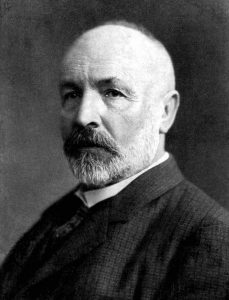# Cardinality and Countably Infinite Sets

by | Jan 12, 2021 | Math Learning

Cardinality is a term used to describe the size of sets. Set A has the same cardinality as set B if a bijection exists between the two sets. We write this as |A| = |B|. One important type of cardinality is called “countably infinite.” A set A is considered to be countably infinite if a bijection exists between A and the natural numbers ℕ. Countably infinite sets are said to have a cardinality of אo (pronounced “aleph naught”).

Remember that a function f is a bijection if the following condition are met:

1. It is injective (“1 to 1”): f(x)=f(y)⟹x=y

2. It is surjective (“onto”): for all b in B there is some a in A such that f(a)=b.

A set is a bijection if it is both a surjection and an injection.

Example 1. Show that the set of integers ℤ is countably infinite.

To show that ℤ is countably infinite, we must find a bijection between ℕ and ℤ, i.e. we need to find a way to match up each element of ℕ to a unique element of ℤ, and this function must cover each element in ℤ.

We can start by writing out a pattern. One pattern we can use is to count down starting at 0, then going back and “picking up” each positive integer. This follows the pattern {0, -1, 1, -2, 2, -3, 3…}. We match to ℕ to ℤ as follows:

Notice that each even natural number is matched up to it’s half. It follows the function f(n) = n/2.

The odd numbers follow the function f(n) = -(n+1)/2

We can write this as a piecewise function as:, if n is even, if n is odd

Now we need to check if our function is a bijection.

Injectivity: Suppose the function is not injective. Then there exist some natural numbers x and y such that f(x)=f(y) but x≠y.

For even integers, x/2 = y/2 ⟹ x=y

For odd integers, Then (-x+1)/2 = -(y+1)/2 ⟹ x=y

These are contradictions, so the function is injective.

Surjectivity: Suppose the function is not surjective. Then there is some integer k such that there is no n in ℕ for which f(n) = k.

For k≥0, k=n/2 ⟹2k=n ⟹ n is an even number in ℕ

For k<0, k=-(n+1)/2 ⇒ -2k-1 = n ⇒ n is an odd number in ℕ

These are contradictions, so the function is surjective.

Since f is both injective and surjective, it is a bijection. Therefore, |ℤ| = |N| =אo. ∎

This result is often surprising to students because the set ℕ is contained in the set ℤ.

Example 2. For A = {2n | n is a number in ℕ}, show that |A| = |ℤ| (the set of integers has the same cardinality as the set of even natural numbers).

We can either find a bijection between the two sets or find a bijection from each set to the natural numbers. Since we already found a bijection from ℤ to ℕ in the previous example, we will now find a bijection from A to ℕ.

One function that will work is f(n) = n/2. Checking that it is a bijection is very similar to Example 1.

Since |A| = |ℕ| and |ℤ| = |ℕ|, then |A| = |ℤ| = אo.∎

There are many sets that are countably infinite, ℕ, ℤ, 2ℤ, 3ℤ, nℤ, and ℚ. All of the sets have the same cardinality as the natural numbers ℕ. Some sets that are not countable include ℝ, the set of real numbers between 0 and 1, and ℂ.Georg Cantor was a pioneer in the field of set theory and was the first to explore countably infinite sets

Featured Bundles